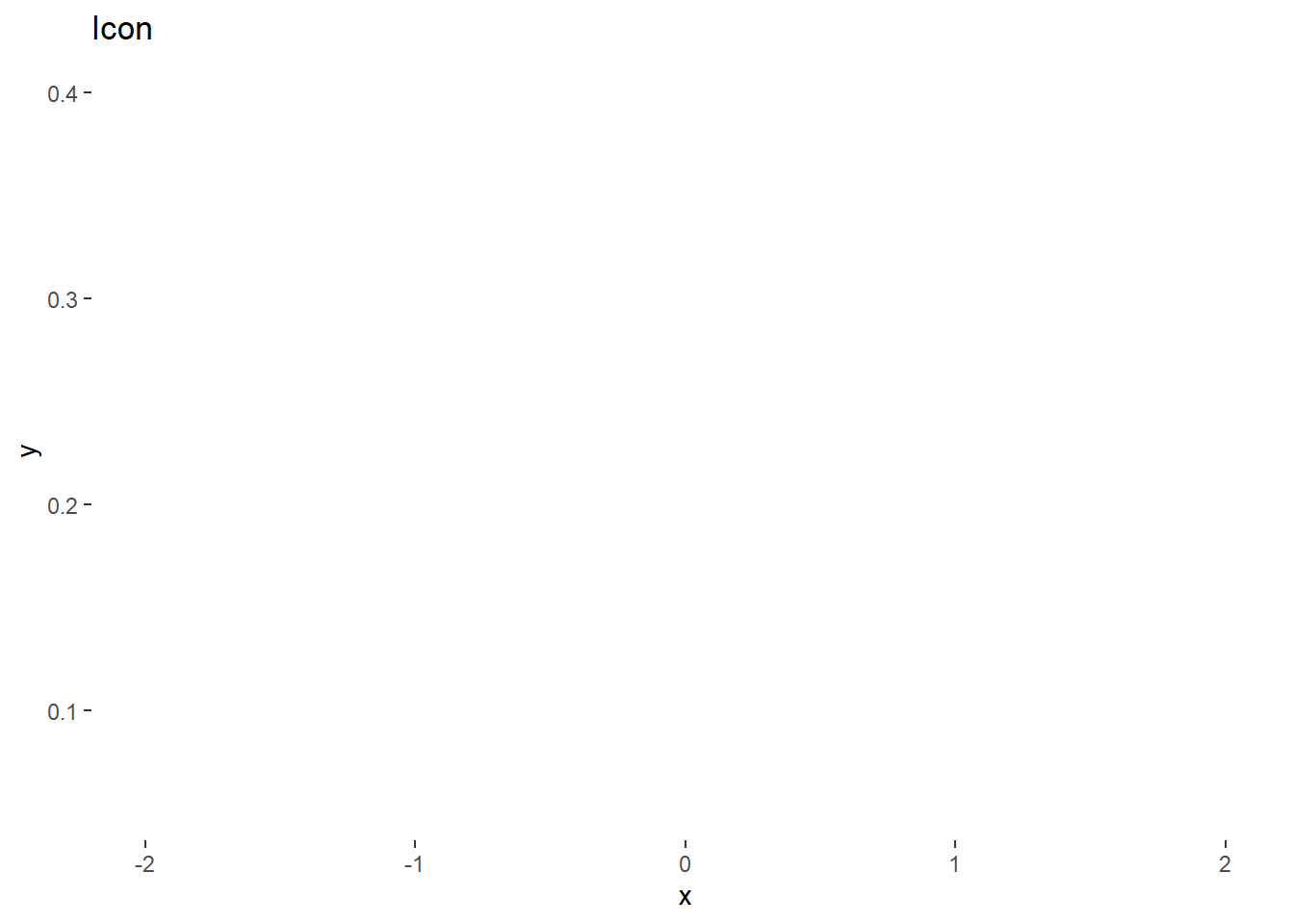Want to share your content on R-bloggers? click here if you have a blog, or here if you don't.Icon
noun
A person or thing regarded as a representative symbol or as worthy of veneration.
A symbol or graphic representation on a screen of a program, option, or window.
from Oxford English Dictionaries

Fontawesome and the noun project along with other icon provides produce and distribute beautiful icons for free use. But sometimes, I would like to alter or create my own tiny 16×16 or 32×32 pixel wide icons without having to learn inkscape or any other svg editor. The figure should have one/two colors on a transparent background.

So first I will try to make an icon of a normal distribution using `ggplot2`. We start with a typical normal distribution on the basic `ggplot2` theme.

``library(ggplot2)``
``## Warning: package 'ggplot2' was built under R version 3.5.1``
``````p <- ggplot(data.frame(x = c(-2, 2)), aes(x)) +
stat_function(fun = dnorm)
p``````In this case, we want a white icon so the curve should be white.

``````p <- ggplot(data.frame(x = c(-2, 2)), aes(x)) +
stat_function(fun = dnorm, color = "white")
p````````p + ggtitle("Icon")``To make the background transparent, we can edit the `panel.background` and `plot.background` elements in `theme.`

``````p + ggtitle("Icon") +
theme(panel.background = element_rect(fill="transparent",colour=NA),
plot.background = element_rect(fill="transparent",colour=NA))``````This doesn’t look very informative so we can just change one of the backgrounds temporarily so we can see the different elements.

``````p + ggtitle("Icon") +
theme(panel.background = element_rect(fill="transparent",colour=NA),
plot.background = element_rect(fill="grey",colour=NA))``````The elements outside the actual plot is controlled by `text`, `line`, and `rect` (as explained here). So we can set all three to white as well.

``````p + ggtitle("Icon") +
theme(panel.background = element_rect(fill="transparent",colour=NA),
plot.background = element_rect(fill="grey",colour=NA),
text = element_text(color = "white"),
line = element_line(color = "white"),
rect = element_rect(fill = "transparent", colour = NA))``````The axis tick marks and grid lines would not be extraneous in an icon and can be removed as well.

``````p + ggtitle("Icon") +
theme(panel.background = element_rect(fill="transparent",colour=NA),
plot.background = element_rect(fill="grey",colour=NA),
text = element_text(color = "white"),
line = element_line(color = "white"),
rect = element_rect(fill = "transparent", colour = NA),
axis.text = element_blank(),
axis.ticks = element_blank(), panel.grid = element_blank())``````We can add back the axis lines with `axis.line`.

``````p + ggtitle("Icon") +
theme(panel.background = element_rect(fill="transparent",colour=NA),
plot.background = element_rect(fill="grey",colour=NA),
text = element_text(color = "white"),
line = element_line(color = "white"),
rect = element_rect(fill = "transparent", colour = NA),
axis.text = element_blank(),
axis.ticks = element_blank(), panel.grid = element_blank(),
axis.line = element_line(color = "white"))``````For the real plot, we want the background to be transparent rather than gray.

``````icon_plot <- p + ggtitle("Icon") +
theme(panel.background = element_rect(fill="transparent",colour=NA),
plot.background = element_rect(fill="transparent",colour=NA),
text = element_text(color = "white"),
line = element_line(color = "white"),
rect = element_rect(fill = "transparent", colour = NA),
axis.text = element_blank(),
axis.ticks = element_blank(), panel.grid = element_blank(),
axis.line = element_line(color = "white"))``````

We can save this resulting plot as both `svg` and `png` using `ggsave`. As `ggsave` uses width and height in inches/centimeters but we would like to produce images of pixel size, we can use the `dpi` parameter to avoid doing any math.

These transparent plots can also work on colored infographics as abstract figures but convey much more detail. since `ggplot2` allows for detailed control of the plot, changing text and line sizes can generate all kinds of

A 72×72 pixel svg.

``ggsave(filename = "icon_72px.svg", icon_plot, dpi=72, width = 1, height = 1)``

A 72×72 pixel png icon. The important argument here is passing the `bg = "transparent"` argument into the `png` device.

``ggsave(filename = "icon_72px.png", icon_plot, dpi=72, width = 1, height = 1, bg = "transparent")``

To actually show the white icon on the page, I’m manually adding a background color.

````On the other hand, if you already have an image you want to convert to an icon, we can use the `magick` package to use `imagemagick` for image processing and scaling.

``library(magick)``
``````## Linking to ImageMagick 6.9.9.14
## Enabled features: cairo, freetype, fftw, ghostscript, lcms, pango, rsvg, webp
## Disabled features: fontconfig, x11``````

We will use the example tiger image from the `magick` vignette.

``````tiger <- image_read_svg('http://jeroen.github.io/images/tiger.svg', width = 400)
print(tiger)``````
``````##   format width height colorspace matte filesize density
## 1    PNG   400    400       sRGB  TRUE        0   72x72``````First, we convert color to two colors only with `image_convert`.

``tiger %>% image_convert(type = "bilevel") ``Since the background is black, and we would like it transparent, we can flip the black and white.

``tiger %>% image_convert(type = "bilevel") %>% image_negate()``And lastly, we can scale to pixel size with `image_scale`.

``tiger %>% image_convert(type = "bilevel") %>% image_negate() %>% image_scale("72x")```imagemagick` has much better capabilities and I feel that saving a full-size plot with `ggsave` and then scaling with `magick` will create better icons than using `ggsave` and specifying dpi as above.